# Did I find out something in my dreams? (I)

One day, I was asleep, and in my dream yesterday, I dreamt that I was playing with factorials. I then got this sudden revelation of a weird formula:

$$\LARGE{\frac{1! × 2! × ... × n!}{1 × 2 × ... × 2n}}$$

Simplifying this, we can get:

$\LARGE{\frac{G(n + 2)}{(2n)!}}$

Where $G(z)$ is the $\text{Barnes G-function}$ and $G(n + 2)$ is the superfactorial

When we substitute $n$ with different values, we gain these numbers:

 $n = 3$ $0.01666…$ $n = 4$ $0.00714285…$ $n = 5$ $0.00952380…$ $n = 6$ $0.0519480…$ $n = 7$ $1.438561…$

I decided to plot this using WolframAlpha, but I do not have the Pro Version, so I plotted a smaller range of values instead (because I was bored a lot...)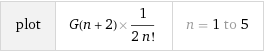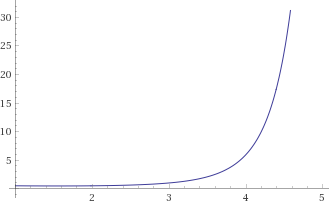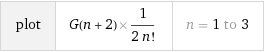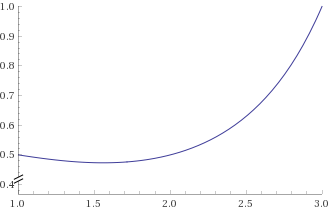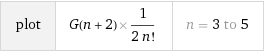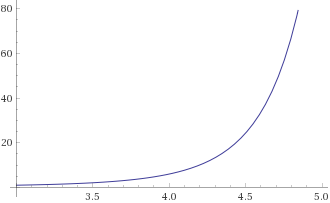Does this graph or number set have any special value to them? I was just bored, but maybe you can find a better explanation for these numbers. I would love to see what random facts you can gain about these weird numbers that came to me in a dream...Note by A Former Brilliant Member
3 months ago

This discussion board is a place to discuss our Daily Challenges and the math and science related to those challenges. Explanations are more than just a solution — they should explain the steps and thinking strategies that you used to obtain the solution. Comments should further the discussion of math and science.

When posting on Brilliant:

• Use the emojis to react to an explanation, whether you're congratulating a job well done , or just really confused .
• Ask specific questions about the challenge or the steps in somebody's explanation. Well-posed questions can add a lot to the discussion, but posting "I don't understand!" doesn't help anyone.
• Try to contribute something new to the discussion, whether it is an extension, generalization or other idea related to the challenge.

MarkdownAppears as
*italics* or _italics_ italics
**bold** or __bold__ bold
- bulleted- list
• bulleted
• list
1. numbered2. list
1. numbered
2. list
Note: you must add a full line of space before and after lists for them to show up correctly
paragraph 1paragraph 2

paragraph 1

paragraph 2

[example link](https://brilliant.org)example link
> This is a quote
This is a quote
    # I indented these lines
# 4 spaces, and now they show
# up as a code block.

print "hello world"
# I indented these lines
# 4 spaces, and now they show
# up as a code block.

print "hello world"
MathAppears as
Remember to wrap math in $$ ... $$ or $ ... $ to ensure proper formatting.
2 \times 3 $2 \times 3$
2^{34} $2^{34}$
a_{i-1} $a_{i-1}$
\frac{2}{3} $\frac{2}{3}$
\sqrt{2} $\sqrt{2}$
\sum_{i=1}^3 $\sum_{i=1}^3$
\sin \theta $\sin \theta$
\boxed{123} $\boxed{123}$

Sort by:

Do these numbers look like something similar, like any other number?

Please give in a small research and let me know...

@Hamza Anushath, Wait! I got it! I know what's special about these numbers! I figured it out!

They all are.....

Decimal numbers (Irrational I think, but could be rational)

Wow! This is my 2nd biggest discovery after I discovered that 1+1 = 2!

LOL, XD!!! LOL, XD!!! LOL, XD!!! LOL, XD!!!

Yes @Yashvardhan Pattanashetti

Plus, they are rational numbers, as they repeat their digits forever

awesome

Maybe you should have written:

I know how to solve it, but this comment section is too small for my proof.

Yours sincerely, Fermat.

- 3 months ago

LOL!! nice!!

Maybe replace the Fermat with @Yashvardhan Pattanashetti's name?

What do you think?

I'd rather have it be Fermat

Ok, then. But good joke, no?

Yup! It was awesome!!

Ok, then.

I guessed you made a discovery in my dreams. I was still awake around $10:30$ when I suddenly thought about new problem series:

Binary clocks

Algebraic binary locks

Basically, I am a walking-talking mathematician as well. @Hamza Anushath, @Yashvardhan Pattanashetti, @Páll Márton

Woah, are you a walking... talking... mathematician!?!

I am just a minor mathematician...

In my sleep, I am talking about being a walking-talking mathematician.

Do you think I am a walking-talking mathematician?

Yes

Ok.

Not the zombie type of walking-talking, though.

We get that. lol

Ok. But what do you think?

Do you think I am a walking-talking mathematician?

Talking-yes, walking, i havent seen you walk, mathematician yes

Ok, then.

What do you think about my new problem series that I mentioned?

I think its a great idea!!

Just thought of two more problem series:

Binary locks

What do you think, @Yashvardhan Pattanashetti?

Nice!! Looking forward to it.

Posting the first hexadecimal lock problem in $5$ mins.

Ok, good luck

Wait - change that to binary locks - hexadecimal locks will never work.

Posting it...

... now

The only thing I was able to link this to was the superfactorial, defined by Neil Sloane and Simon Plouffe to be the product of incrementing factorials (the numerator in your expression). Using the notation for a superfactorial, your expression could simplify to:

$\dfrac{sf(n)}{(2n)!}$

- 3 months ago

Thanks a lot @David Stiff! If you could, could you tell me whether the graph I generated is correct or not...?

You're welcome Hamza!

I wrote some Python code to generate a graph of this expression, and both the numerical values and the graph are identical to those you calculated. I only used the superfactorial form however, not the more general Barnes G-function. I don't think I can post pictures in a reply, but here are the first 10 values I got:

$1, 0.5, 0.08\overline{3}, 0.01\overline{6}, 0.007142857142857143, 0.009523809523809525, 0.05194805194805195, 1.4385614385614385, 241.67832167832168$

I find it interesting that the graph first descends, bottoms out at $n = 4$ and then ascends again, rocketing up at $n = 6$.

- 3 months ago

Thanks a lot once again @David Stiff

P.S. We can post pictures in a comment by uploading it in a note and copying that code and pasting it here, the code starting with ![]

No problem. And thanks for the tip!

- 3 months ago

You can't generate a graph using this function, @David Stiff, @Hamza Anushath

I was just thinking, I could simplify your top equation:

$\frac{n!!}{2n!}$

Try it, @Hamza Anushath

$n!!$ is the double factorial. @Hamza Anushath

@Yajat Shamji, it's not the double Factorial, it is actually a superfactorial...

Oops. But did you try it?

Yes, and very sorry, it brought a wrong value @Yajat Shamji